Next: 4.3 Entropy, Helmholtz Free Energy and Previous: 4.1 The Boltzmann Distribution

# 4.2 The Partition Function

Take-home message: Far from being an uninteresting normalisation constant,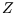is the key to calculating all macroscopic properties of the system!

The normalisation constant in the Boltzmann distribution is also called the partition function: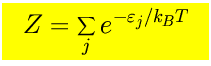where the sum is over all the microstates of the system.

How can a constant be a function? Well for a given system and reservoir, that is fixed temperature, particle number, volume or magnetic field (as appropriate),is a constant. But if the temperature etc are allowed to vary, thenis a function of them: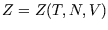or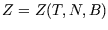. (The dependence on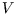or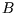comes through the energies of the microstates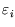)

Why are we emphasising this? Because if we know, we can calculate all macroscopic properties of the system - energy, pressure, magnetisation, entropy...

For instance the average energy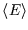(actually an ensemble average) is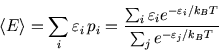The top line is like the bottom line (the partition function) except that each term is multiplied by. We can get the top line from the bottom by differentiating by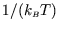''. This is a bit awkward, so we introduce a new symbol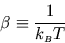giving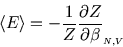or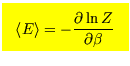(where--contrary to the strict instructions given earlier--we will take it for granted that it is particle number and volume or magnetic field constant that we are holding constant.)

From the energy we can find the heat capacity: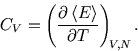We have found the average energy, but there will be fluctuations as heat is randomly exchanged between the system and the heat bath. These are given by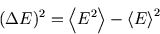It can be shown that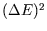is related to the heat capacity,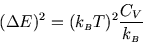For a normal macroscopic system the average energy is of the order of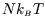and the heat capacity is of the order of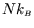. Thus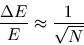For a system of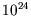atoms,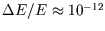and so fluctuations are unobservable. There is no practical difference between and isolated system of energy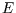and one in contact with a heat bath at the same temperature.

References

• Mandl 2.5
• Bowley and Sánchez 5.2
• Kittel and Kroemer 3

SubsectionsNext: 4.3 Entropy, Helmholtz Free Energy and Previous: 4.1 The Boltzmann Distribution
Judith McGovern 2004-03-17